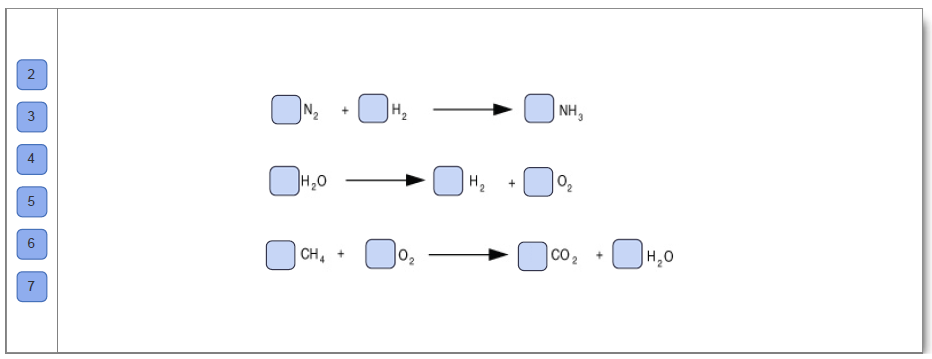# Problem: In the PhET simulation click on the Make Ammonia radio button in the bottom of the screen. Balance the given chemical equation by changing the coefficients placed before each compound. To display more helpful information, select an option from the Tools dropdown menu in the upper right. When a reaction is balanced correctly a yellow face will appear with a smile. Repeat this task with the reactions Separate Water and Combust Methane.Drag the appropriate labels to their respective targets. Leave the target empty for coefficient of 1.Balancing chemical equationsWhen balancing equations, subscripts can never be changed because this reflects a change in the molecular structure; for example, changing CO2 to CO results in a different molecule.To balance an equation, the coefficients are changed so that the number of each type of atom on the reactant side of the equation equals the number of each type of atom on the product side of the equation.Start by balancing atoms that appear in the most complex structures and in the most structures first. Balance elements that occur by themselves last. If a fractional coefficient is present in the chemical equation, then multiply all of the coefficients by the denominator of that fraction to clear the fraction.

###### FREE Expert SolutionView Complete Written Solution
###### Problem Details

In the PhET simulation click on the Make Ammonia radio button in the bottom of the screen. Balance the given chemical equation by changing the coefficients placed before each compound. To display more helpful information, select an option from the Tools dropdown menu in the upper right. When a reaction is balanced correctly a yellow face will appear with a smile. Repeat this task with the reactions Separate Water and Combust Methane.

Drag the appropriate labels to their respective targets. Leave the target empty for coefficient of 1.Balancing chemical equations

When balancing equations, subscripts can never be changed because this reflects a change in the molecular structure; for example, changing CO2 to CO results in a different molecule.

To balance an equation, the coefficients are changed so that the number of each type of atom on the reactant side of the equation equals the number of each type of atom on the product side of the equation.

Start by balancing atoms that appear in the most complex structures and in the most structures first. Balance elements that occur by themselves last. If a fractional coefficient is present in the chemical equation, then multiply all of the coefficients by the denominator of that fraction to clear the fraction.